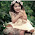-->

## Tuesday, April 14, 2015

### Linux Date Injection into Hive

This week I found myself needing to generate a table in Hive that used today's date in the output location. Basically I was running a daily report and wanted it to automatically send the output to the appropriate bucket on S3.

To accomplish this, I used a combination of embedded Linux commands and Hive variables.

First, in your Hive query, you need to turn on variable substitution:

set hive.variable.substitute=true;

Next, in your Hive query you can have an expression substituted for the variable value. For instance, you can create a table like this:

CREATE EXTERNAL TABLE IF NOT EXISTS my_table
(
values STRING,
)
ROW FORMAT DELIMITED FIELDS TERMINATED BY '\t'
LINES TERMINATED BY '\n'
LOCATION 's3://mybucket/${hiveconf:DATE_VARIABLE}'; The Hive syntax for a variable is${hiveconf:VARNAME}When calling Hive, you can give it a variable by using the -hiveconf VARNAME=VALUE syntax. For instance:

hive -hiveconf DATE_VARIABLE=$(date +y=%Y/m=%m/d=%d/h=%H/) -f query.sql Notice that the value of the variable in the above query is$(date +y=%Y/m=%m/d=%d/h=%H/). This is the syntax for telling Linux to execute the command inside the $( ) and return the value. You can also use backticks (  ) instead of$( ). Essentially the date command will run, returning a date string like y=2015/m=04/d=13 and assign that to the Hive variable. That variable will then be substituted in the Hive query and build a custom table location.

Super handy.

1.2.